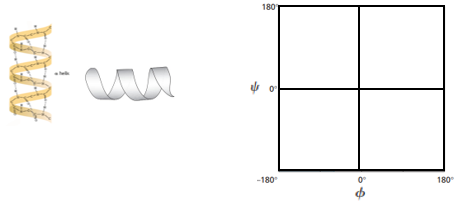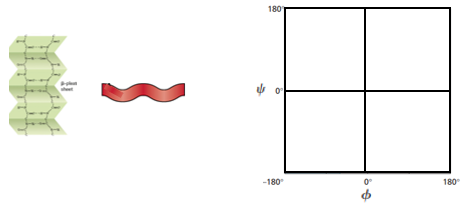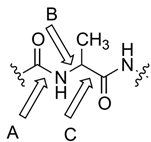## Biochemistry

Learn the toughest concepts covered in Biochemistry with step-by-step video tutorials and practice problems by world-class tutors

4. Protein Structure

# Ramachandran Plot

1
concept

## Ramachandran Plot7m
Play a video:
in this video, we're gonna begin our discussion on Rama Condron plots. So in our last lesson video, we said that peptide bond rotation is limited and restricted due to its partial double bond character. But bond rotations specifically around the Alfa Carbon bonds are still possible, even though the peptide bond rotation is restricted. And it turns out that there are three bond rotation angles that you guys should be familiar with, and they're symbolized with these Greek letters and so down below. In our example, we're gonna distinguish between these three bond angles in this peptide that's being shown. And so in this peptide we have a free amino group on the far left and on the far right. What we have is a little squiggly line which represents a continuation of the peptide. So we're not being shown the C terminal end and we're on Lee being shown a small little snippet of our peptide, and so notice that the are groups of our peptide here are in blue. And so the first amino acid residue has a hydrogen as its our group, which means that we have a glazing amino acid residue and the second amino acid residue has a metal group, as it's our group, which means that we have an Alan een amino acid residue. And so to distinguish between these three bond angles, the first bond angle that you guys should know is the five bond angle. And FYI is symbolized with the Greek letter Phi, and it represents the rotation angles around the Alfa carbon nitrogen bond in the backbone. And so, taking a look at our Allan, an amino acid residue down below in our peptide noticed that the Alfa Carbon is this carbon that's here, which is attached to the our group. And so the Alfa Carbon bonded to the nitrogen is going to represent the fi bond. And so you can see that this bond right here is the fi Bond and Fi represents the bond rotation angles around the fi bond, so the second bond rotation angle that you guys should know is the sigh Bond and Sigh is symbolized with the Greek letter sigh, and it represents rotation angles around the Alfa Carbon Carbonnel group carbon bond in the backbone. And so, taking a look at our Allen and amino acid residue again, here is our Alfa Carbon and the Carbonnel Group. Carbon is over here. And so the bond between the Alfa Carbon and the Carbonnel Group Carbon is the side bond and Sigh represents the bond rotation angles around the side bond. And so the last bond rotation angle that you guys should be familiar with is Omega, and Omega is symbolized with the Greek letter omega, and it represents rotation angle of the peptide bond. And so, looking below at our peptide, we can see that the peptide bond is in red over here and notice that it has partial double bond character with this dotted line. And Omega is shown here to represent the bond rotation angles around the peptide bond. Now we know that Omega and the bond rotation angles around the peptide bond is severely limited and restricted because of the partial double bond character. And because it's restricted, it's not really interesting toe plot Omega on a Rama Condron plot. And so it turns out that Omega is actually excluded from a Rama Condra plot, and when we're plotting a Rama Condron plot, we only focus on the fi and the Sai bond rotation angles. And so, looking over here, at our other figure. What we have is a ball and stick figure of a peptide and noticed that the Alfa Carbon is this black ball that's here in the center. And so the bonds that are around the Alfa Carbon are all able to still rotates. So bond rotations around the Alfa Carbon are still possible. And so that's why you see that the Alfa Carbon nitrogen bonds of this blue ball over here represents the nitrogen. We said that the Alfa Carbon nitrogen bond is the fi bond. So this bond here in green is analogous to this bond over here in green, which is the five bond. And then the side bond is going to be this bond over here and yellow between the Alfa Carbon and the Carbonnel group carbon. And so you can see that these arrows that are being shown are indicating that these bonds are able to freely rotate, whereas the peptide bond, which would be, uh, this bond over here in red and this bond over here, these bonds are restricted and limited, and that's why we see the plane behind them that indicates the uh, peptide group that is plainer and So what we what this means because we're mainly gonna be focusing on fi and Side bonds is that we need a way to distinguish between the fire and the side bonds. And so what helps me is that when you actually write out five, um, you'll see that the H here really looks like a larger version of a lower case end. And the end here reminds you of the nitrogen atom. And so that can tell you that thief I Bond is really just the Alfa Carbon nitrogen bond. And that's exactly what Fi is all about. It's the Alfa Carbon nitrogen bond. And again, both the fi and the SAI have the Alfa Carbon. So really distinguishes them is the nitrogen and the carbonnel group carbon. And so, if you can remember that this little H here looks like a lower case N, which reminds you of the nitrogen atom. Then you'll remember that fi as the Alfa Carbon nitrogen bond Now for Cy. On the other hand, when you draw the Greek letter side, you literally draw sideways, see with a line through it, and so the sideways see can remind you of a carbon and So the side bond is all about the Alfa Carbon carbon bonds. So there's Onley carbons that are involved, and so the sigh here can remind you of the side bond. And so, if you can remember these memory tools, you'll be able to distinguish between the fi and the Sai bonds. And so the last thing I want to leave you guys off with is that within a peptide, each individual amino acid residue has its own fi and side bonds. And so if we take a look at our glazing amino acid residue over here, notice that it's Alfa Carbon is right here. And so the Alfa Carbon nitrogen bond, which would be this bond right here, is going to be the fi bond. Just like we said before, Alfa Carbon Nitrogen is the five bond. So what we can do is we can draw on our Greek symbol, Fi right there. And so the Alfa Carbon carbon bond over here is going to be the Cy Bond. And so this bond here in yellow is gonna be the Cy Bond so we can draw in our Greek symbol side. And then, of course, the peptide bonds are always going to be. So this pond right here is going to be the peptide bond, and that's gonna represent Omega, which we can draw in here as well. And so moving forward, we're gonna talk, Maura about Rama Condron plots, and we'll be able to get, ah, lot more practice understanding Fi inside bond angles after we talk about Rama condom plots. And so I'll see you guys in our next video, where we'll be able to get a little bit of practice distinguishing between fi and side bond angles, so I'll see you guys there.
2
concept

## Ramachandran Plot6m
Play a video:
3
Problem

A Ramachandran plot shows:

4
Problem

Why are some regions of a Ramachandran plot shaded while others are unshaded? Circle all true answers.

5
Problem

The predominate structure in α-keratin, a mammalian protein that makes up large portions of hair & nails, is the α-helix. Mark the approximate locations on a Ramachandran plot you might expect to find φ and ψ angles for α-keratin amino acid residues.6
Problem

The principal component of silk is the protein fibroin, which is a classic example of β-sheet structure. Mark the approximate locations on a Ramachandran plot you might expect to find φ and ψ angles for silk amino acid residues.